@TOC

• 示例：

~~~cpp

# include

int main() { int array[] = { 4,1,8,5,3,7,0,9,2,6 }; // 預設按照小於比較，排出來結果是升序 std::sort(array, array + sizeof(array) / sizeof(array)); for (int i = 0; i < 10; i++) { cout << array[i] << " "; }cout << endl; // 如果需要降序，需要改變元素的比較規則 std::sort(array, array + sizeof(array) / sizeof(array), greater()); for (int i = 0; i < 10; i++) { cout << array[i] << " "; }cout << endl; return 0; } ~~~

• 效果：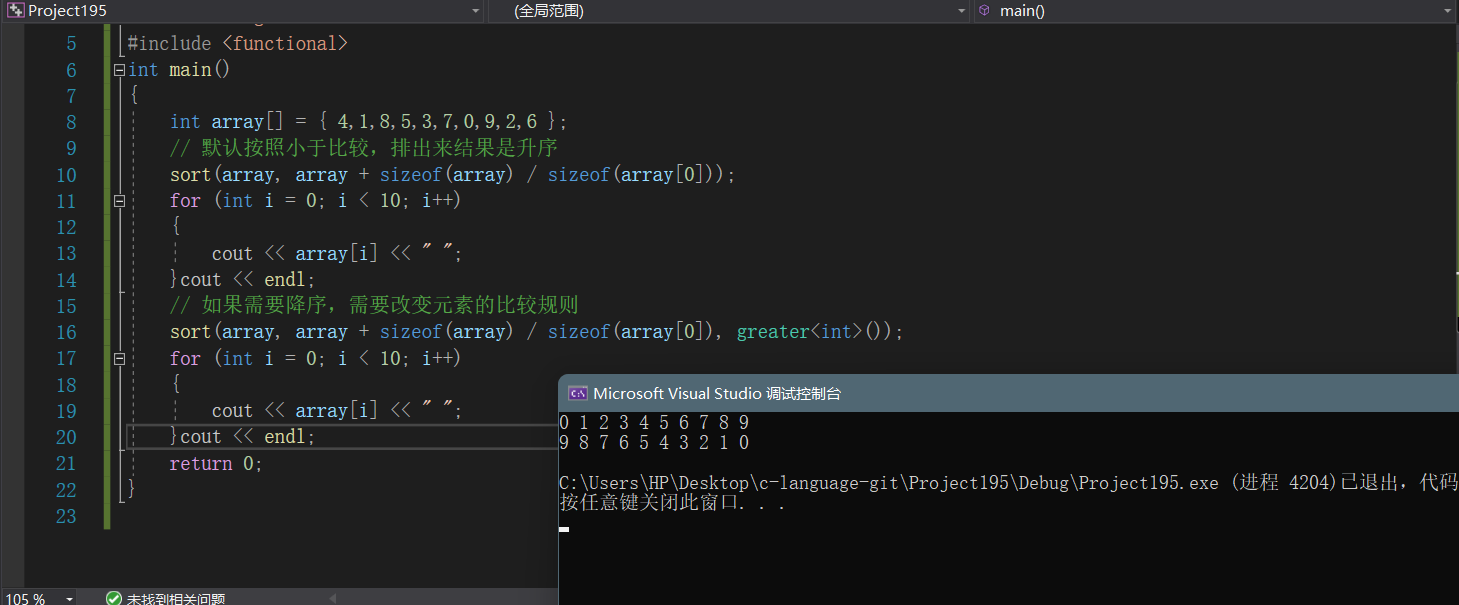• 示例：

~~~cpp struct Goods { string _name; double _price; }; struct Compare { bool operator()(const Goods& gl, const Goods& gr) { return gl._price <= gr._price; } }; int main() { Goods gds[] = { { "蘋果", 2.1 }, { "香蕉", 3 }, { "橙子", 2.2 }, {"菠蘿", 1.5} }; sort(gds, gds + sizeof(gds) / sizeof(gds), Compare()); for (int i = 0; i < 4; i++) cout << gds[i]._name << ":"<<gds[i]._price<<" "; cout << endl; return 0; } ~~~

• 效果：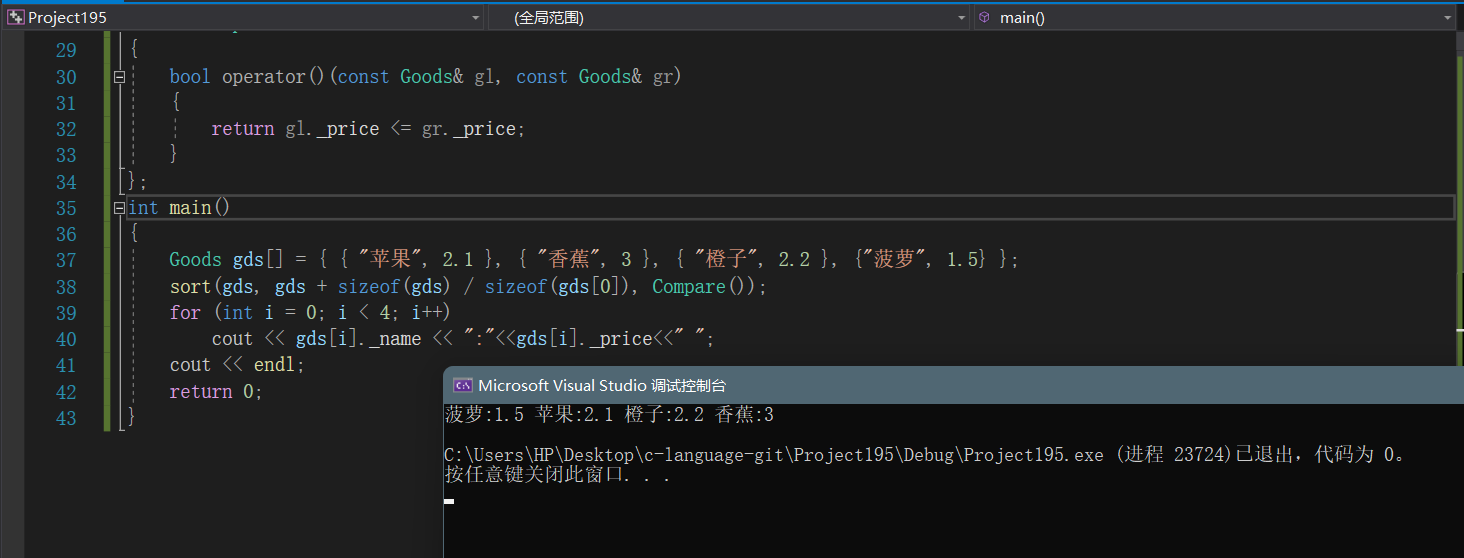• 概念及引入：

• 示例：

~~~cpp int main() { Goods gds[] = { { "蘋果", 2.1 }, { "香蕉", 3 }, { "橙子", 2.2 }, {"菠蘿", 1.5} }; sort(gds, gds + sizeof(gds) / sizeof(gds), ->bool { return l._price < r._price; }); return 0; } ~~~

## 2、lambda表示式語法

• lambda表示式書寫格式：

~~~cpp [capture-list] (parameters) mutable -> return-type { statement } ~~~

• lambda表示式各部分說明：
1. [capture-list] :

1. (parameters)：

1. mutable：

1. ->returntype：

1. {statement}：

• 示例：

~~~cpp int main() { // 最簡單的lambda表示式, 該lambda表示式沒有任何意義 [] {}; // 省略引數列表和返回值型別，返回值型別由編譯器推導為int int a = 3, b = 4; [=]{ return a + 3; }; // 省略了返回值型別，無返回值型別 auto fun1 = & { b = a + c; }; fun1(10); cout << a << " " << b << endl; // 各部分都很完善的lambda函式 auto fun2 = =, &b->int {return b += a + c; }; cout << fun2(10) << endl; // 複製捕捉x int x = 10; auto add_x = xmutable { x = 2; return a + x; };//傳值捕捉修改需要mutable修飾 auto add_x1 = &x{ x = 2; return a + x; };//引用捕捉不用 cout << add_x(10) << endl; cout << x << endl; cout << add_x1(10) << endl; cout << x << endl; return 0; } ~~~

• 效果：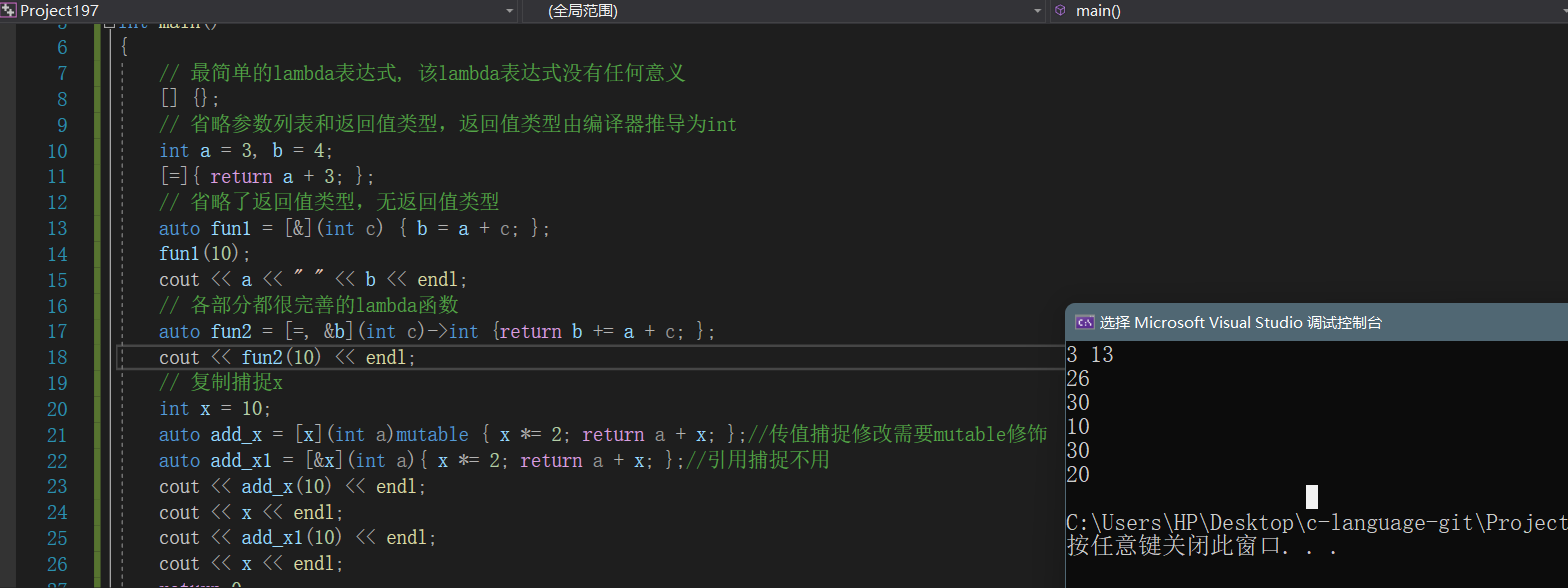## 3、捕獲列表說明

• 概念：

• 使用方式：

~~~cpp [var]：表示值傳遞方式捕捉變數var [=]：表示值傳遞方式捕獲所有父作用域中的變數(包括this) [&var]：表示引用傳遞捕捉變數var [&]：表示引用傳遞捕捉所有父作用域中的變數(包括this) [this]：表示值傳遞方式捕捉當前的this指標 ~~~

• 注意：
1. 父作用域指包含lambda函式的語句塊

2. 語法上捕捉列表可由多個捕捉項組成，並以逗號分割：比如：[=, &a, &b]：以引用傳遞的方式捕捉變數a和b，值傳遞方式捕捉其他所有變數 [&，a, this]：值傳遞方式捕捉變數a和this，引用方式捕捉其他變數

3. 捕捉列表不允許變數重複傳遞，否則就會導致編譯錯誤：比如：[=, a]：=已經以值傳遞方式捕捉了所有變數，捕捉a重複

4. 在塊作用域以外的lambda函式捕捉列表必須為空；在塊作用域中的lambda函式僅能捕捉父作用域中區域性變數

5. lambda表示式之間不能相互賦值，即使看起來型別相同

• 示例：

~~~cpp void (*PF)(); int main() { auto f1 = []{cout << "hello world" << endl; }; auto f2 = []{cout << "hello world" << endl; }; //f1 = f2; // 編譯失敗--->提示找不到operator=() // 允許使用一個lambda表示式拷貝構造一個新的副本 auto f3(f2); f3(); // 可以將沒有捕獲任何變數的lambda表示式賦值給相同型別的函式指標 PF = f2; PF(); return 0; } ~~~

• 解釋：

Lambda是實現了函式呼叫運算子的匿名類(anonymous class)。對於每一個Lambda，編譯器建立匿名類，並定義相應的資料成員儲存Lambda捕獲的變數。沒有捕獲變數的Lambda不包含任何含成員變數。一個沒有任何成員變數(包括沒有虛擬函式表指標)的型別，在空指標上呼叫成員函式也不會有任何的問題，因為它的成員函式不會通過this指標訪問記憶體。當Lambda向函式指標的轉換時，編譯器為Lambda的匿名類實現函式指標型別轉換運算子

## 4、函式物件與lambda表示式

• 示例：

~~~cpp class Rate { public: Rate(double rate): _rate(rate) {} double operator()(double money, int year) { return money * _rate * year; } private: double _rate; }; int main() { // 函式物件 double rate = 0.49; Rate r1(rate); r1(10000, 2); // lamber auto r2 = =->double{return montyrateyear; }; r2(10000, 2); return 0; } ~~~

• 說明：

• 示圖：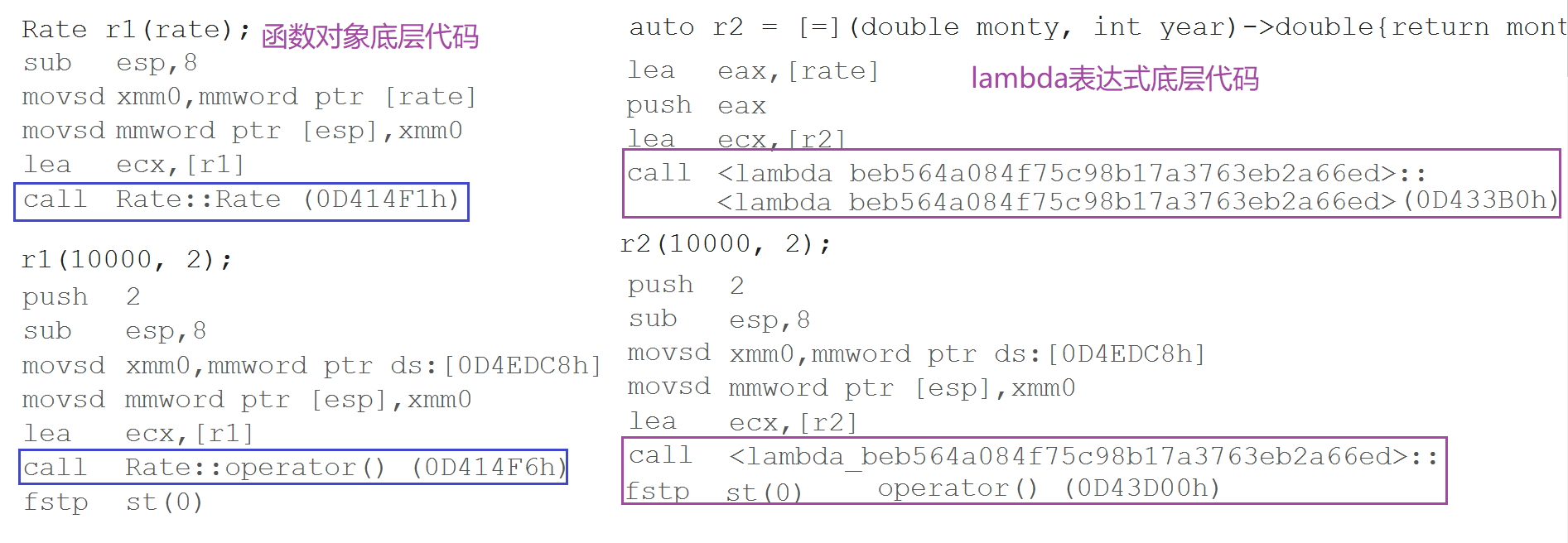# 二、包裝器

## 1、function包裝器

• 概念：

function包裝器也叫作介面卡，C++中的function本質是一個類模板，也是一個包裝器

• 存在的問題：
1. 函式指標型別太複雜，不方便使用和理解
2. 仿函式型別是一個類名，沒有指定呼叫引數和返回值，得去看operator()的實現才能看出來
3. lambda表示式在語法層，看不到型別，只能在底層看到其型別，基本都是lambda_uuid
• 示例：

~~~cpp template T useF(F f, T x) { static int count = 0; cout << "count:" << ++count << endl; cout << "count:" << &count << endl; return f(x); } double f(double i) { return i / 2; } struct Functor { double operator()(double d) { return d / 3; } }; int main() { // 函式名 cout << useF(f, 11.11) << endl; // 函式物件 cout << useF(Functor(), 11.11) << endl; // lamber表示式 cout << useF(->double { return d / 4; }, 11.11) << endl; return 0; } ~~~

• 效果：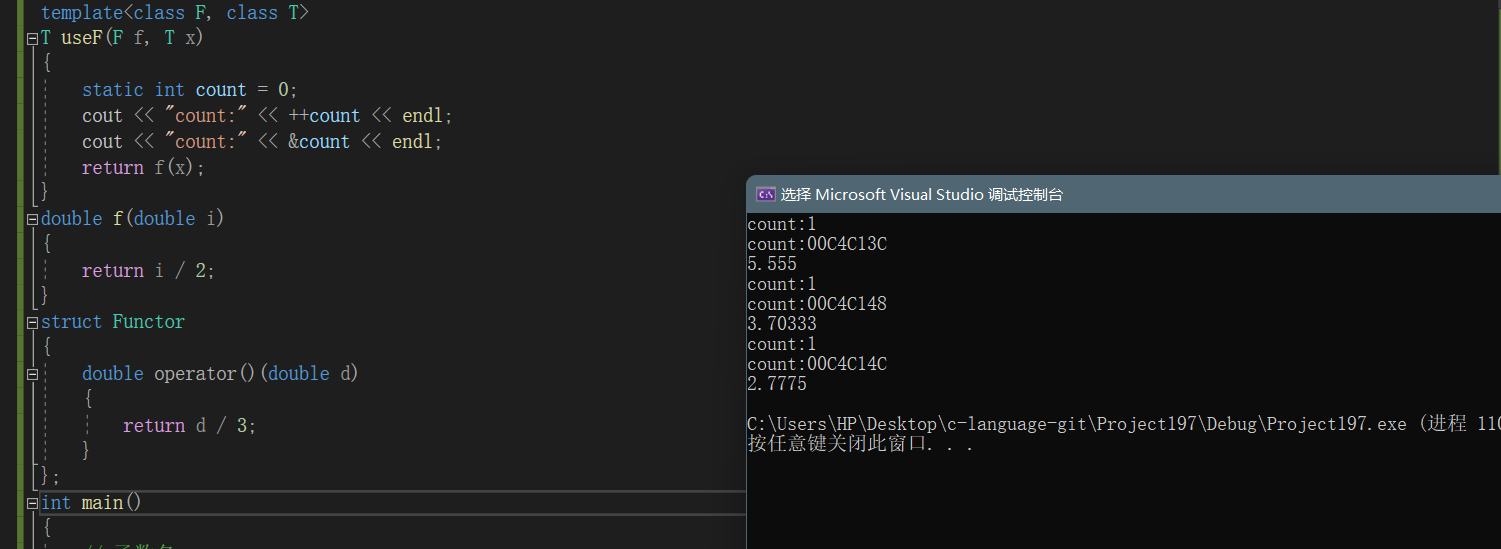• 包裝器原型：

~~~cpp // 類模板原型如下 template function; // undefined template class function; ~~~

Ret: 被呼叫函式的返回型別

Args…：被呼叫函式的形參

~~~cpp

# include

int f(int a, int b) { return a + b; } struct Functor { int operator() (int a, int b) { return a + b; } }; class Plus { public: static int plusi(int a, int b) { return a + b; } double plusd(double a, double b) { return a + b; } }; int main() { // 函式名(函式指標) std::function func1 = f; cout << func1(1, 2) << endl; // 函式物件 std::function func2 = Functor(); cout << func2(1, 2) << endl; // lamber表示式 std::function func3 = { return a + b; }; cout << func3(1, 2) << endl; // 類的成員函式 std::function func4 = Plus::plusi; cout << func4(1, 2) << endl; std::function func5 = &Plus::plusd;//對於普通成員的包裝一定要加上&，需要通過指標進行呼叫成員函式 cout << func5(Plus(), 1.1, 2.2) << endl;//傳入類物件，通過物件進行呼叫 return 0; } ~~~

• 效果：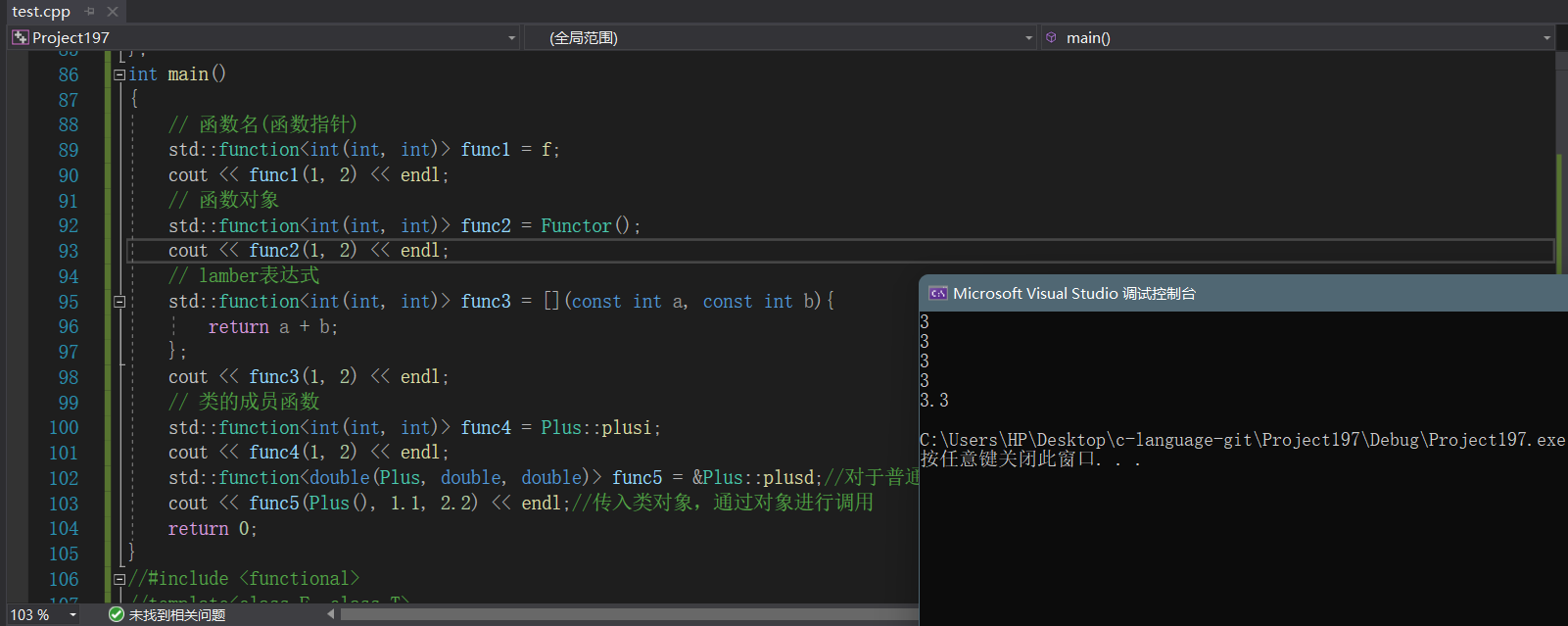• 包裝器解決模板例項化多份的問題：

~~~cpp

# include

template T useF(F f, T x) { static int count = 0; cout << "count:" << ++count << endl; cout << "count:" << &count << endl; return f(x); } double f(double i) { return i / 2; } struct Functor { double operator()(double d) { return d / 3; } }; int main() { //將多個可呼叫型別進行封裝成相同型別，便於統一呼叫 // 函式名 std::function func1 = f; cout << useF(func1, 11.11) << endl; // 函式物件 std::function func2 = Functor(); cout << useF(func2, 11.11) << endl; // lamber表示式 std::function func3 = ->double { return d /4; }; cout << useF(func3, 11.11) << endl; return 0; } ~~~

• 效果：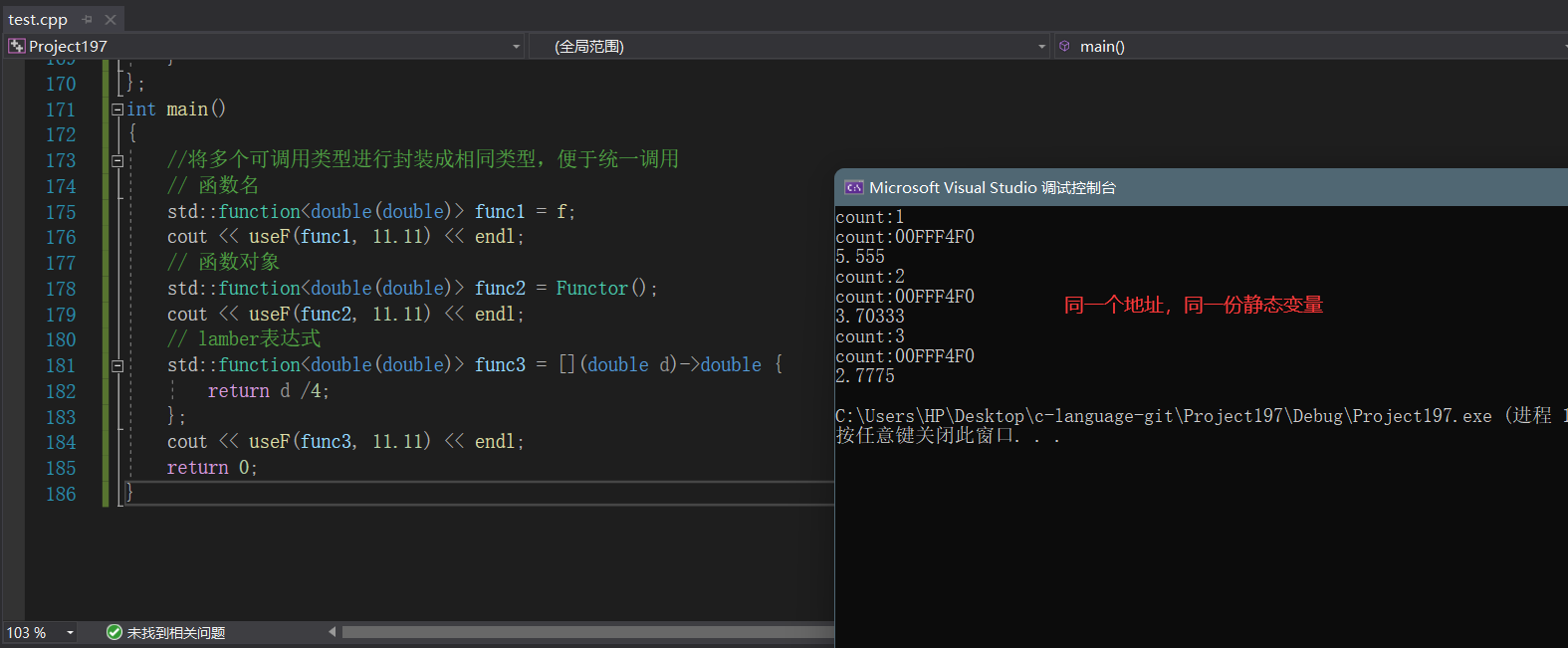## 2、bind

• 概念：
1. std::bind函式定義在標頭檔案中，是一個函式模板，它就像一個函式包裝器(介面卡)，接受一個可呼叫物件（callable object），生成一個新的可呼叫物件來“適應”原物件的引數列表
2. 一般而言，我們用它可以把一個原本接收N個引數的函式fn，通過繫結一些引數，返回一個接收M個（M可以大於N，但這麼做沒什麼意義）引數的新函式；同時，使用std::bind函式還可以實現引數順序調整等操作
• 示例：

~~~cpp

# include

int Plus(int a, int b) { return a + b; } class Sub { public: int sub(int a, int b) { return a - b; } static int sub1(int a, int b) { return a - b; } }; int main() { //普通函式的繫結 //表示繫結函式plus 引數分別由呼叫 func1 的第一，二個引數指定(placeholders用來表示引數位佔位) std::function func1 = std::bind(Plus, placeholders::_1,placeholders::_2); //auto func1 = std::bind(Plus, placeholders::_1, placeholders::_2);直接使用auto識別型別

``````//表示繫結函式 plus 的第一，二引數為： 1， 2
auto func2 = std::bind(Plus, 1, 2);
cout << func1(1, 2) << endl;
cout << func2(2,3) << endl;//func2();也可以不用傳引數-因為引數已經繫結好了，傳入的引數沒有實際的作用

//類函式的繫結
//類的成員函式必須通過類的物件或者指標呼叫，因此在bind時，bind的第一個引數的位置來指定一個類的實列、指標或引用。
Sub s;
// 繫結成員函式
std::function<int(int, int)> func3 = std::bind(&Sub::sub, s,placeholders::_1, placeholders::_2);
// 引數調換順序
std::function<int(int, int)> func4 = std::bind(&Sub::sub, s,placeholders::_2, placeholders::_1);
std::function<int(int, int)> func5 = std::bind(Sub::sub1,placeholders::_2, placeholders::_1);//靜態成員函式的繫結-不需要類的示例指標或引用
cout << func3(1, 2) << endl;
cout << func4(1, 2) << endl;
cout << func5(1, 2) << endl;
return 0;
``````

} ~~~

• 效果：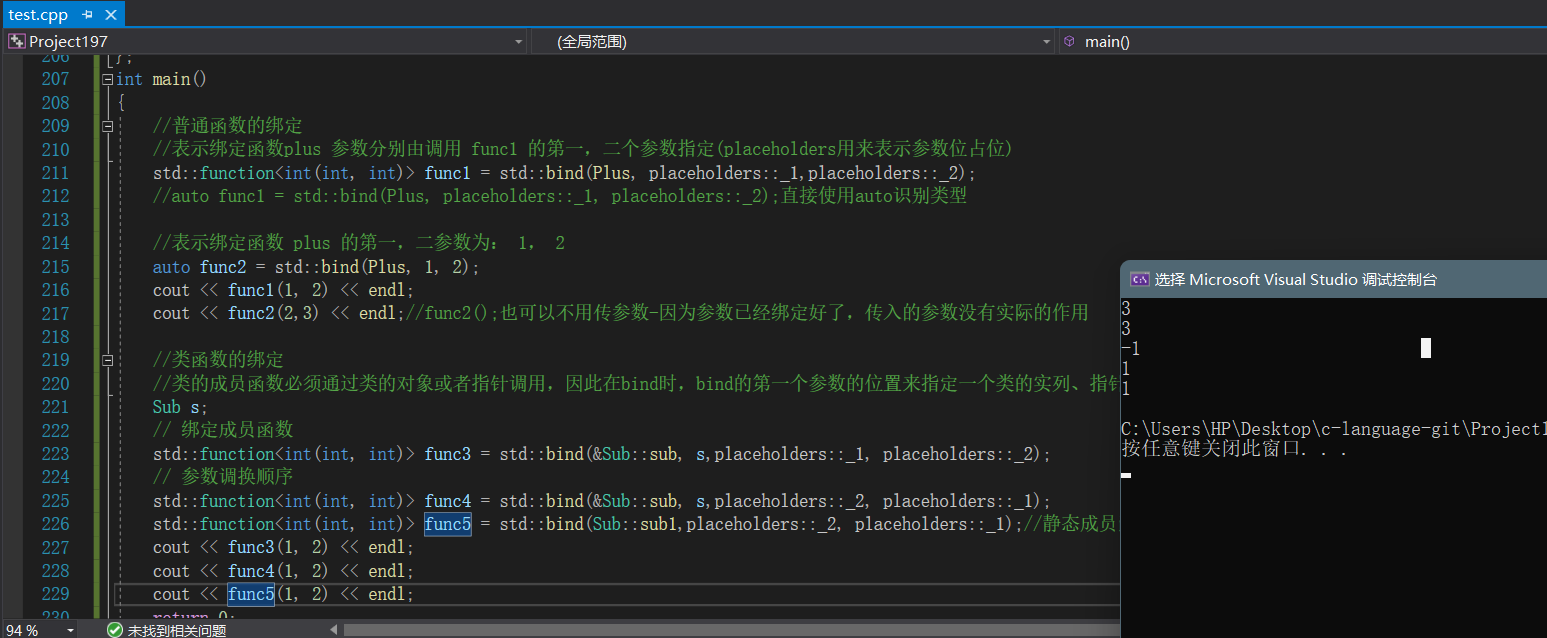• 總結：

bind是對包裝的可呼叫型別的進一步封裝，可以根據自己的需要進行調整引數的資料及位置，繫結類物件能有優化成員函式的包裝使用，更加符合使用習慣

# 三、執行緒庫

## 1、執行緒的概念及使用

1. 在C++11之前，涉及到多執行緒問題，都是和平臺相關的，比如windows和linux下各有自己的介面，這使得程式碼的可移植性比較差
2. C++11中最重要的特性就是對執行緒進行支援了，使得C++在並行程式設計時不需要依賴第三方庫，而且在原子操作中還引入了原子類的概念

• 執行緒常用介面：

| 函式名 | 功能 | | ----------------------------- | ------------------------------------------------------------ | | thread() | 構造一個執行緒物件，沒有關聯任何執行緒函式，即沒有啟動任何執行緒 | | thread(fn, args1, args2, ...) | 構造一個執行緒物件，並關聯執行緒函式fn，args1，args2，...為執行緒函式的 引數 | | get_id() | 獲取執行緒id | | jionable() | 執行緒是否還在執行，joinable代表的是一個正在執行中的執行緒。 | | jion() | 該函式呼叫後會阻塞住執行緒，當該執行緒結束後，主執行緒繼續執行 | | detach() | 在建立執行緒物件後馬上呼叫，用於把被建立執行緒與執行緒物件分離開，分離 的執行緒變為後臺執行緒，建立的執行緒的"死活"就與主執行緒無關 |

• 注意：
1. 執行緒是作業系統中的一個概念，是程序中的一個執行分支，執行緒物件可以關聯一個執行緒，用來控制執行緒以及獲取執行緒的狀態

2. 當建立一個執行緒物件後，沒有提供執行緒函式，該物件實際沒有對應任何執行緒

• 示例：

~~~cpp

# include

int main() { std::thread t1;//空執行緒 cout << t1.get_id() << endl; return 0; } ~~~

• 對應結構體的定義：

~~~cpp // vs下檢視 typedef struct { / thread identifier for Win32 / void _Hnd; / Win32 HANDLE */ unsigned int _Id; } _Thrd_imp_t; ~~~

1. 當建立一個執行緒物件後，並且給執行緒關聯執行緒函式，該執行緒就被啟動，與主執行緒一起執行
• 執行緒函式一般情況下可按照以下三種方式提供：

• 函式指標

• lambda表示式
• 函式物件
• 示例：

~~~cpp

# include

using namespace std;

void ThreadFunc(int a) { cout << "Thread1" << a << endl; } class TF { public: void operator()() { cout << "Thread3" << endl; } }; int main() { // 執行緒函式為函式指標 thread t1(ThreadFunc, 10); // 執行緒函式為lambda表示式 thread t2( { cout << "Thread2" << endl; }); // 執行緒函式為函式物件 TF tf; thread t3(tf); t1.join(); t2.join(); t3.join(); cout << "Main thread!" << endl; return 0; } ~~~

• 效果：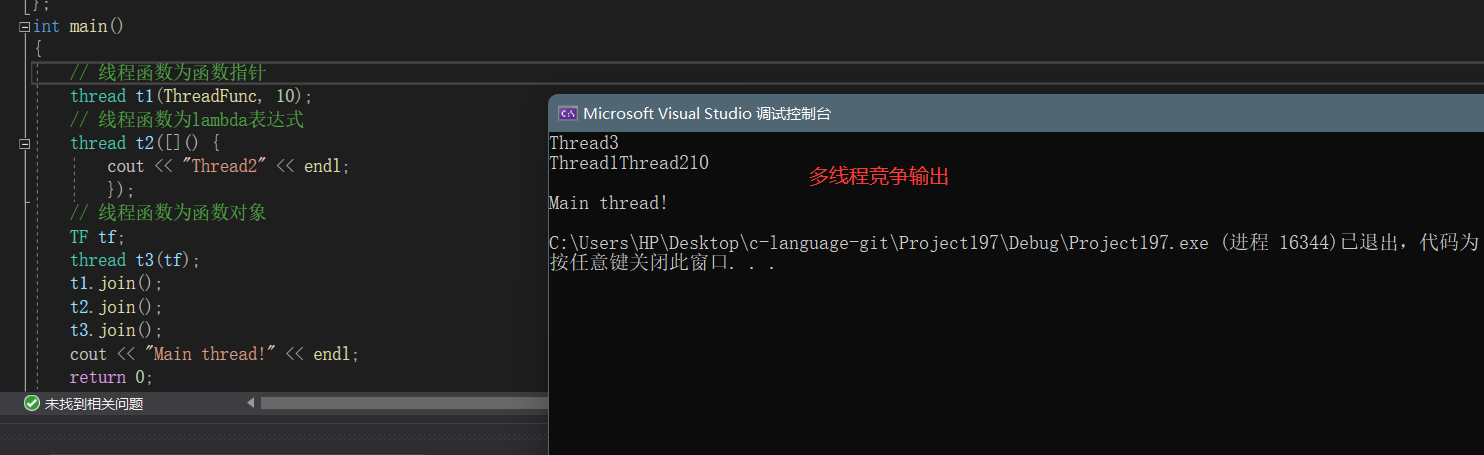• 無效的執行緒：

• 採用無參建構函式構造的執行緒物件

• 執行緒物件的狀態已經轉移給其他執行緒物件
• 執行緒已經呼叫jion或者detach結束
• 面試題：併發與並行的區別
1. 併發指的是多個事情，在同一時間段內同時發生了；並行指的是多個事情，在同一時間點上同時發生了
2. 併發的多個任務之間是互相搶佔資源的；並行的多個任務之間是不互相搶佔資源的，只有在多CPU的情況中才會發生並行

• 示例：

~~~cpp

# include

using namespace std; void Func1(int& x) { x += 10; return; } void Func2(int x) { x += 10; return; } int main() { int a = 10; // 線上程函式中對a修改，不會影響外部實參，因為：執行緒函式引數雖然是引用方式，但其實際引用的是執行緒棧中的拷貝 // vs2019會報錯-對於引用的引數這麼傳入 //thread t1(Func1, a); //t1.join(); //cout << a << endl; // 如果想要通過形參改變外部實參時，必須藉助std::ref()函式 thread t2(Func1, ref(a)); t2.join(); cout << a << endl; // 地址的拷貝 thread t3(Func2, &a); t3.join(); cout << a << endl; return 0; } ~~~

• 效果：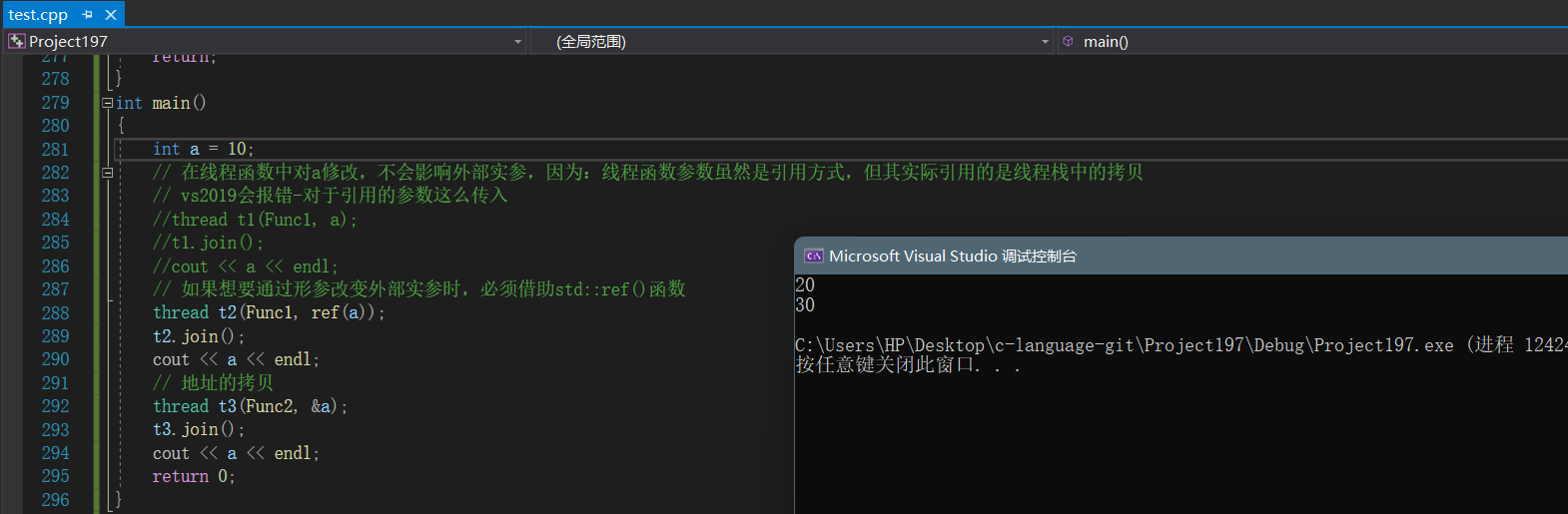• 注意：

• 示例：

~~~cpp

# include

using namespace std; class A { public: void Func1(int x) { cout << x << endl; } static void Func2(int x) { cout << x << endl; } }; int main() { A a; //普通成員函式需要傳入類的例項或者指標 thread t1(&A::Func1, a, 10); t1.join(); //靜態成員函式則不用 thread t2(&A::Func2, 10); t2.join(); return 0; } ~~~

• 效果：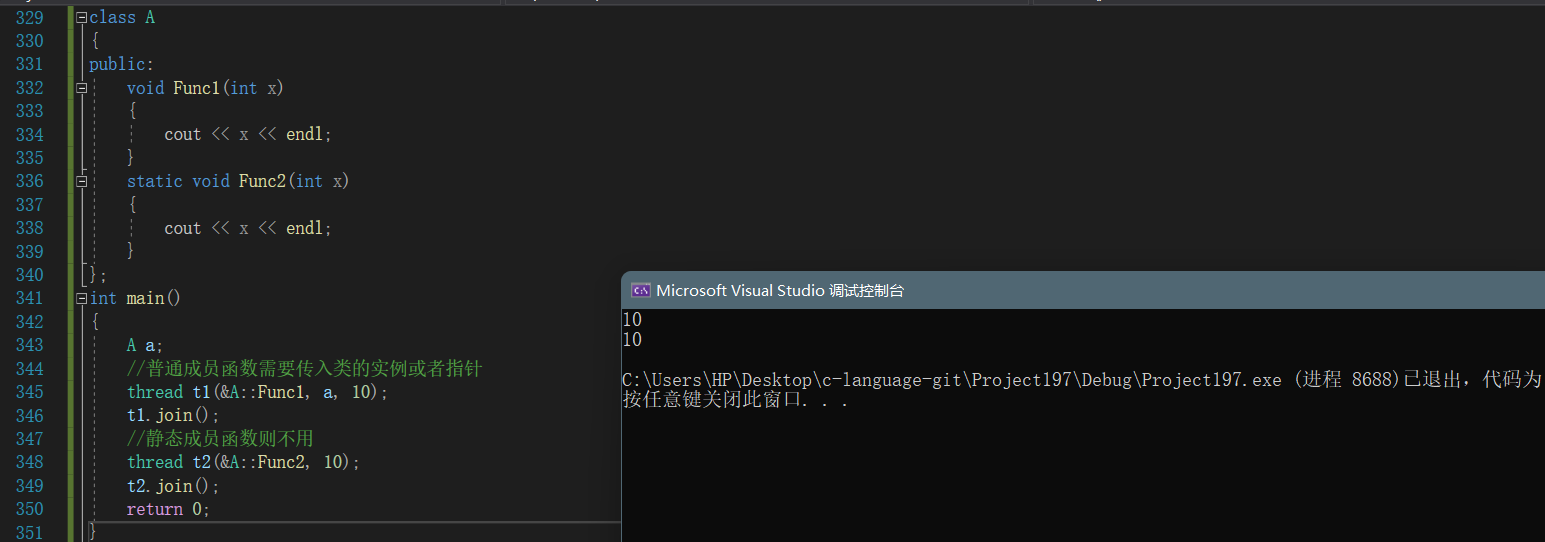• 示例：

~~~cpp

# include

using namespace std;

unsigned long sum = 0L; void fun(size_t num) { for (size_t i = 0; i < num; ++i) sum++; } int main() { cout << "Before joining,sum = " << sum << std::endl; thread t1(fun, 10000000); thread t2(fun, 10000000); t1.join(); t2.join(); cout << "After joining,sum = " << sum << std::endl; return 0; } ~~~

• 效果：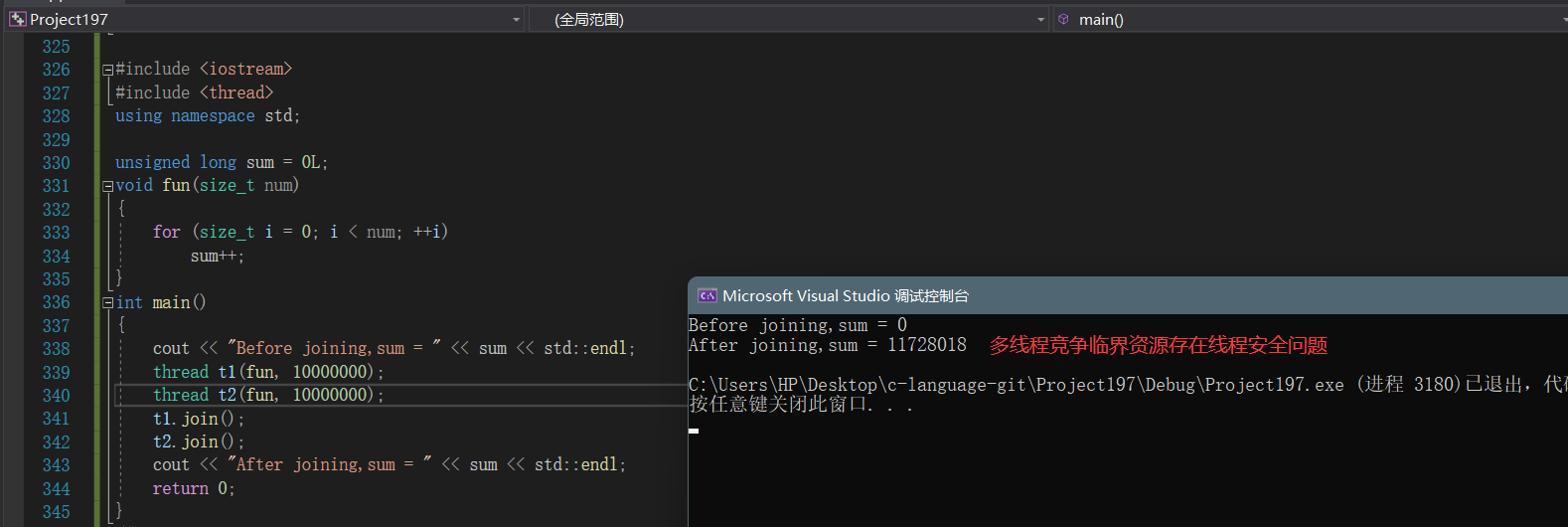C++98中傳統的解決方式：可以對共享修改的資料可以加鎖保護

• 示例：

~~~cpp

# include

using namespace std;

std::mutex m; unsigned long sum = 0L; void fun(size_t num) { for (size_t i = 0; i < num; ++i) { m.lock(); sum++; m.unlock(); } } int main() { cout << "Before joining,sum = " << sum << std::endl; thread t1(fun, 10000000); thread t2(fun, 10000000); t1.join(); t2.join(); cout << "After joining,sum = " << sum << std::endl; return 0; } ~~~

• 效果：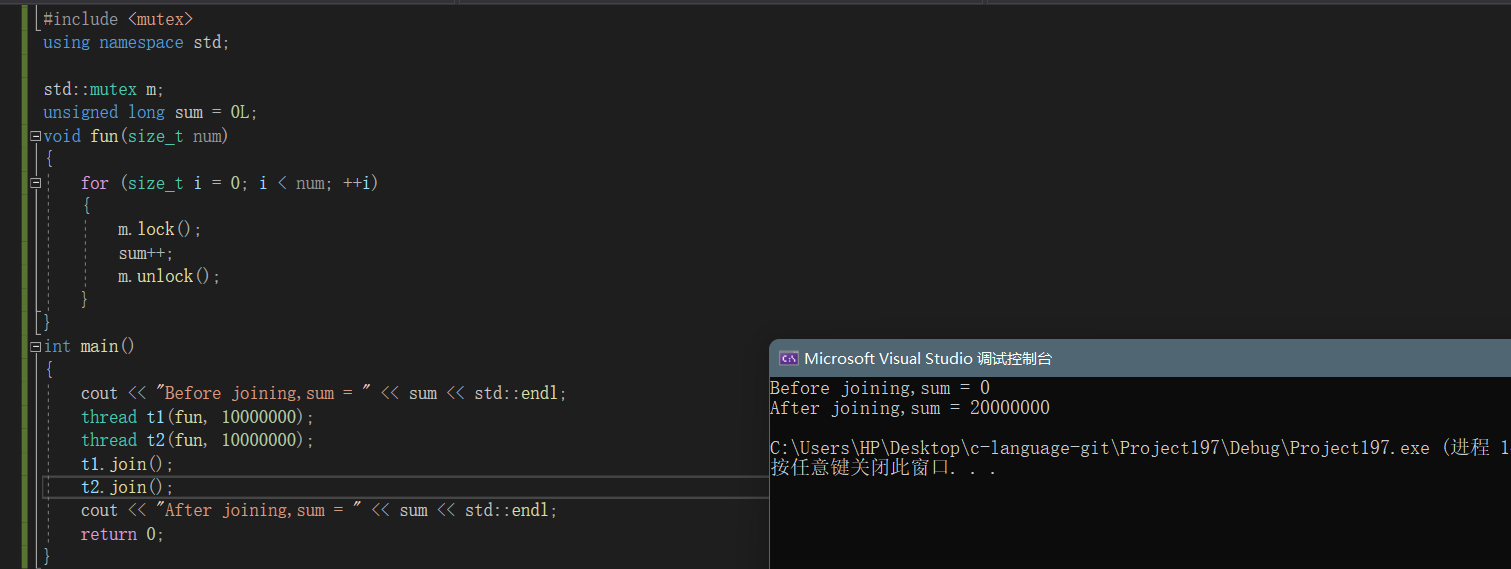• 加鎖缺陷：

• 示圖：原子操作型別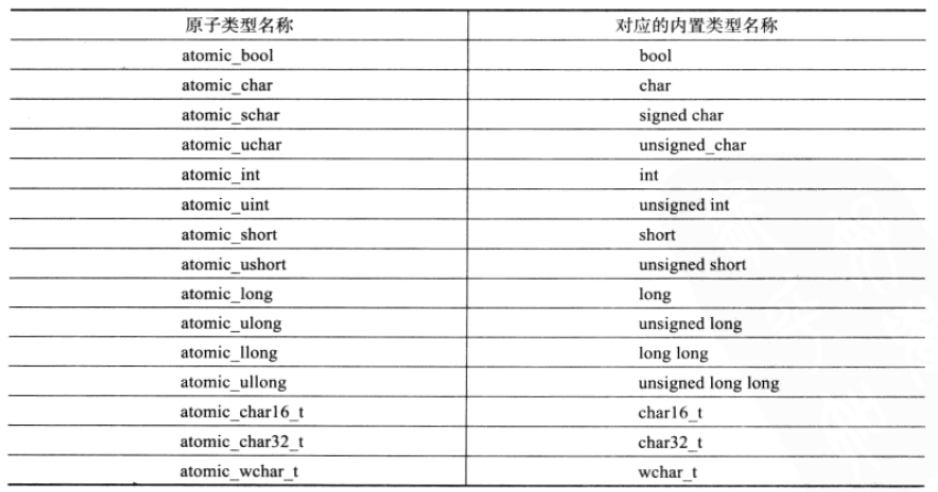• 示例：

~~~cpp

# include

using namespace std;

# include

atomic_long sum{ 0 }; void fun(size_t num) { for (size_t i = 0; i < num; ++i) sum++; // 原子操作 } int main() { cout << "Before joining, sum = " << sum << std::endl; thread t1(fun, 1000000); thread t2(fun, 1000000); t1.join(); t2.join(); cout << "After joining, sum = " << sum << std::endl; return 0; } ~~~

• 效果：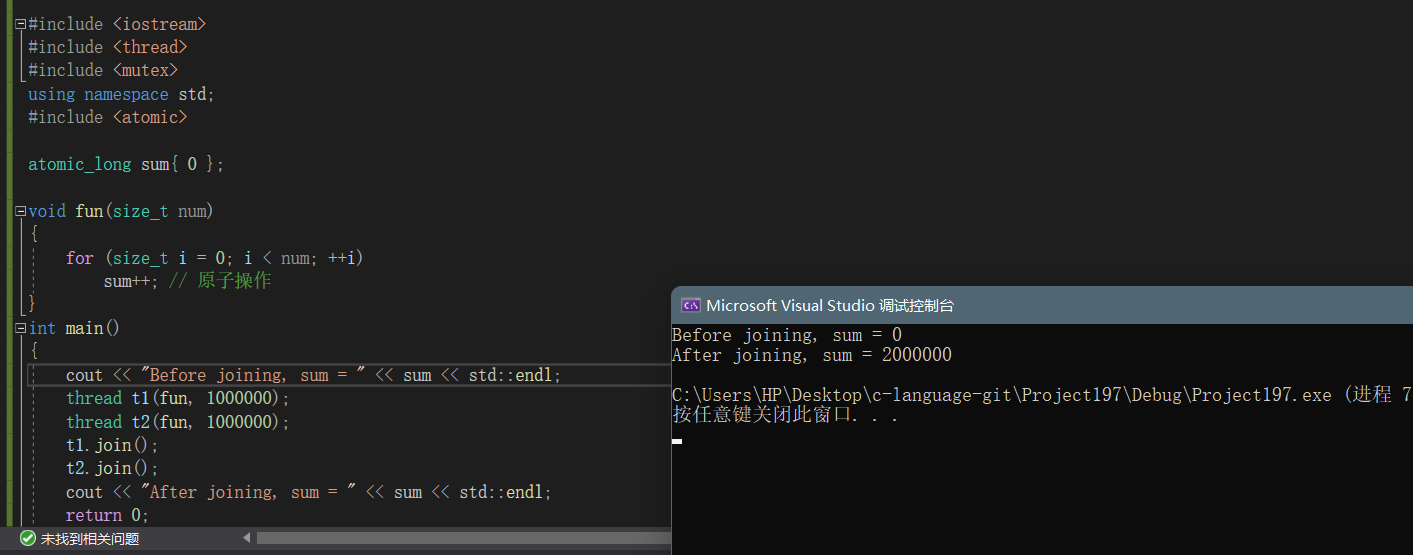• 注意：

~~~cpp int main() { thread t1(fun, 1000000); thread t2(fun, 1000000); t1.join(); t2.join(); //printf("%d\n", sum);vs2019存在型別不匹配問題 //解決方式 //1. printf("%ld\n", sum.load()); //2. cout << sum << endl; //3. printf("%ld\n", (long)sum); return 0; } ~~~

• atomic類模板:

~~~cpp atmoic t; // 宣告一個型別為T的原子型別變數t ~~~

• 注意：

• 示例：

~~~cpp

# include

int main() { atomic a1(0); //atomic a2(a1); // 編譯失敗 atomic a2(0); //a2 = a1; // 編譯失敗 return 0; } ~~~

## 4、lock_guard與unique_lock

• 概念及引入：
1. 在多執行緒環境下，如果想要保證某個變數的安全性，只要將其設定成對應的原子型別即可，即高效又不容易出現死鎖問題

2. 但是有些情況下，我們可能需要保證一段程式碼的安全性，那麼就只能通過鎖的方式來進行控制，鎖控制不好時，可能會造成死鎖 ，最常見的比如在鎖中間程式碼返回，或者在鎖的範圍內拋異常

3. 因此：C++11採用RAII的方式對鎖進行了封裝，即lock_guard和unique_lock

### 1、mutex的種類

• 在C++11中，Mutex總共包了四個互斥量的種類：

• std::mutex

C++11提供的最基本的互斥量，該類的物件之間不能拷貝，也不能進行移動

• mutex最常用的三個函式：

| 函式名 | 函式功能 | | ---------- | ------------------------------------------------------------ | | lock() | 上鎖：鎖住互斥量 | | unlock() | 解鎖：釋放對互斥量的所有權 | | try_lock() | 嘗試鎖住互斥量，如果互斥量被其他執行緒佔有，則當前執行緒也不會被阻塞 |

• 執行緒函式呼叫lock()時可能會發生以下三種情況：
1. 如果該互斥量當前沒有被鎖住，則呼叫執行緒將該互斥量鎖住，直到呼叫 unlock之前，該執行緒一直擁有該鎖
2. 如果當前互斥量被其他執行緒鎖住，則當前的呼叫執行緒被阻塞住
• 執行緒函式呼叫try_lock()時可能會發生以下三種情況：
1. 如果當前互斥量沒有被其他執行緒佔有，則該執行緒鎖住互斥量，直到該執行緒呼叫 unlock 釋放互斥量
2. 如果當前互斥量被其他執行緒鎖住，則當前呼叫執行緒返回 false，而並不會被阻塞掉
1. std::recursive_mutex
1. 其允許同一個執行緒對互斥量多次上鎖（即遞迴上鎖），來獲得對互斥量物件的多層所有權，釋放互斥量時需要呼叫與該鎖層次深度相同次數的 unlock()
2. 除此之外，std::recursive_mutex 的特性和 std::mutex 大致相同
1. std::timed_mutex
1. 比 std::mutex 多了兩個成員函式，try_lock_for()，try_lock_until() , try_lock_for()
2. 接受一個時間範圍，表示在這一段時間範圍之內執行緒如果沒有獲得鎖則被阻塞住（與std::mutex 的 try_lock() 不同，try_lock 如果被呼叫時沒有獲得鎖則直接返回false），如果在此期間其他執行緒釋放了鎖，則該執行緒可以獲得對互斥量的鎖，如果超時（即在指定時間內還是沒有獲得鎖），則返回 false
3. try_lock_until()接受一個時間點作為引數，在指定時間點未到來之前執行緒如果沒有獲得鎖則被阻塞住，如果在此期間其他執行緒釋放了鎖，則該執行緒可以獲得對互斥量的鎖，如果超時（即在指定時間內還是沒有獲得鎖），則返回 false
1. std::recursive_timed_mutex

recursive_mutex和timed_mutex的結合

### 1、lock_guard

~~~cpp template class lock_guard { public: // 在構造lock_gard時上鎖 explicit lock_guard(_Mutex& _Mtx) : _MyMutex(_Mtx) { _MyMutex.lock(); } lock_guard(_Mutex & _Mtx, adopt_lock_t) : _MyMutex(_Mtx) {} // 在析構lock_gard時解鎖 ~lock_guard() _NOEXCEPT { _MyMutex.unlock(); } lock_guard(const lock_guard&) = delete; lock_guard& operator=(const lock_guard&) = delete; private: _Mutex& _MyMutex; }; ~~~

• 解釋：

lock_guard類模板主要是通過RAII的方式，對其管理的互斥量進行了封裝，在需要加鎖的地方，只需要用上述介紹的任意互斥體例項化一個lock_guard，呼叫建構函式成功上鎖，出作用域前，lock_guard物件要被銷燬，呼叫解構函式自動解鎖，可以有效避免死鎖問題

• lock_guard的缺陷：

### 3、unique_lock

• 概念及介紹：
1. 與lock_gard類似，unique_lock類模板也是採用RAII的方式對鎖進行了封裝，並且也是以獨佔所有權的方式管理mutex物件的上鎖和解鎖操作，即其物件之間不能發生拷貝
2. 在構造(或移動(move)賦值)時，unique_lock 物件需要傳遞一個 Mutex 物件作為它的引數，新建立的unique_lock 物件負責傳入的 Mutex 物件的上鎖和解鎖操作。使用以上型別互斥量例項化unique_lock的物件時，自動呼叫建構函式上鎖，unique_lock物件銷燬時自動呼叫解構函式解鎖，可以很方便的防止死鎖問題
3. 與lock_guard不同的是，unique_lock更加的靈活，提供了更多的成員函式： 上鎖/解鎖操作：lock、try_lock、try_lock_for、try_lock_until和unlock 修改操作：移動賦值、交換(swap：與另一個unique_lock物件互換所管理的互斥量所有權)、釋放(release：返回它所管理的互斥量物件的指標，並釋放所有權) 獲取屬性：owns_lock(返回當前物件是否上了鎖)、operator bool()(與owns_lock()的功能相同)、mutex(返回當前unique_lock所管理的互斥量的指標)

## 5、兩個執行緒交替列印奇數偶數

• 錯誤示例：使用普通的條件變數
1. 先讓列印偶數執行緒獲取到所資源，然後在條件變數下等待並將鎖資源釋放
2. 列印奇數獲取到鎖進行列印，列印後先喚醒在條件變數下等待的執行緒，再等待在並釋放鎖資源
3. 再列印偶數執行緒被喚醒並競爭到鎖資源，進行列印...

~~~cpp

# include

using namespace std;

int main() { int i = 1; int j = 2; bool flg = true; mutex mtx; condition_variable cv; //存在時間片切出去的問題 thread t2(&j, &mtx, &cv { while (j <= 100) { std::unique_lock lock(mtx); cv.wait(lock); cout << std::this_thread::get_id() << ":" << j << endl; j += 2; cv.notify_one(); } }); thread t1(&i, &mtx, &cv { while (i <= 100) { std::unique_lock lock(mtx); cout << std::this_thread::get_id() << ":" << i << endl; i += 2; cv.notify_one(); cv.wait(lock); } }); t1.join(); t2.join(); return 0; } ~~~

• 問題示例：

• 效果：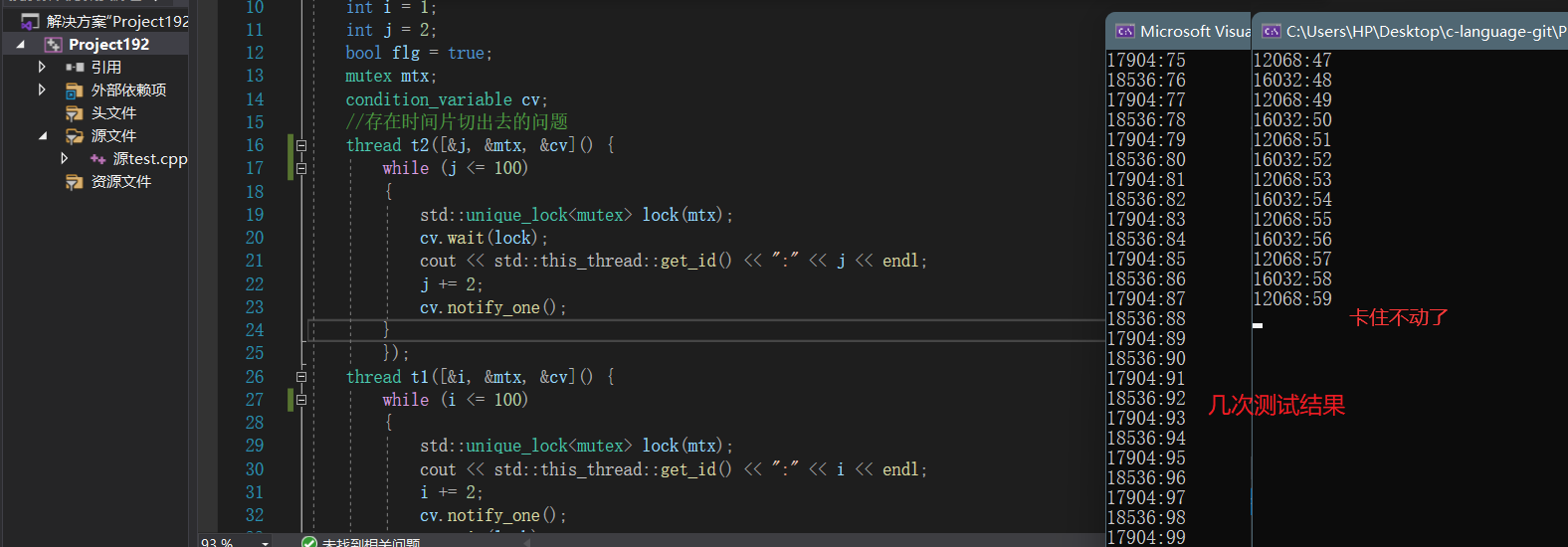• 正確示例：

~~~cpp

# include

using namespace std;

int main() { int i = 1; int j = 2; bool flg = true; mutex mtx; condition_variable cv; //正確寫法 thread t1(&i, &mtx, &cv,&flg { while (i <= 100) { std::unique_lock lock(mtx); cv.wait(lock, &flg { return flg; });//根據條件判斷是否需要進行阻塞等待 cout << std::this_thread::get_id() << ":" << i << endl; i+=2; flg = false;//更改條件變數-使得另一個執行緒執行，該執行緒會等待住 cv.notify_one();//進行喚醒等待條件變數下的執行緒 } }); thread t2(&j, &mtx, &cv, &flg { while (j <= 100) { std::unique_lock lock(mtx); cv.wait(lock, &flg { return !flg; }); cout << std::this_thread::get_id() << ":" << j << endl; j += 2; flg = true; cv.notify_one(); } }); t1.join(); t2.join(); return 0; } ~~~

• 效果：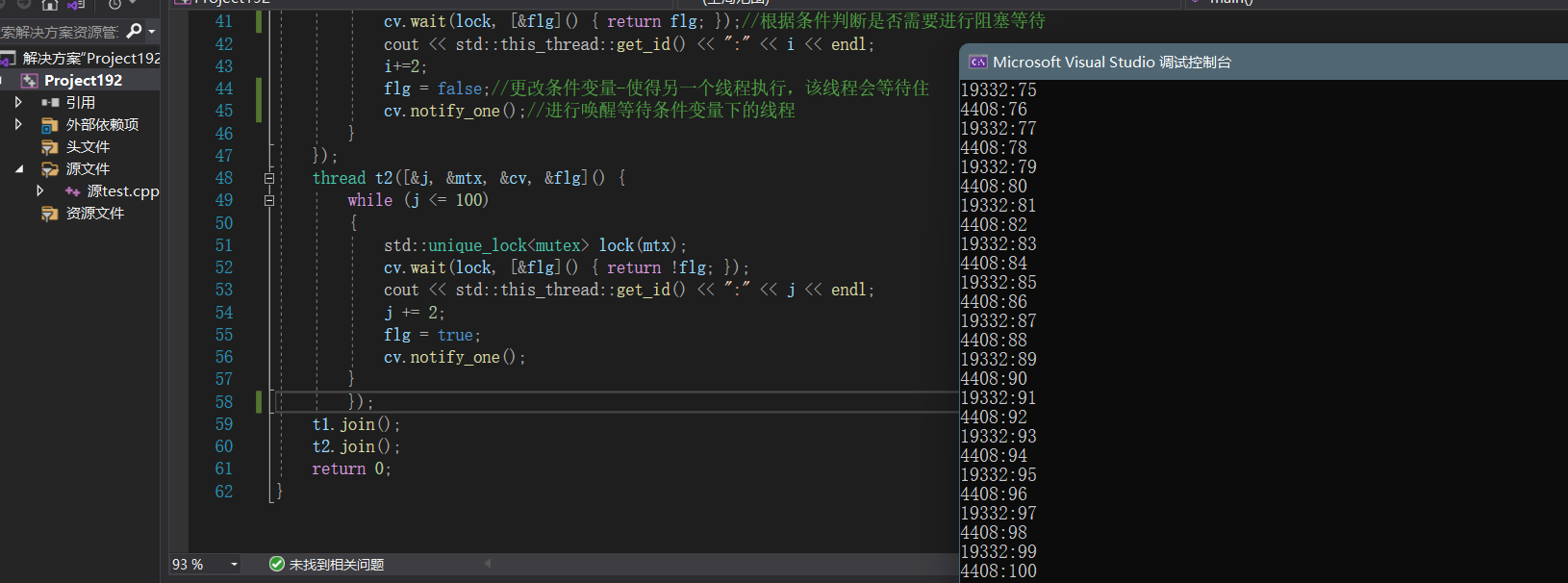~~~cpp

# include

using namespace std;

int main() { int i = 1; int j = 2; bool flg = true; mutex mtx; condition_variable cv; //正確寫法 thread t1(&i, &mtx, &cv,&flg { while (i <= 100) { std::unique_lock lock(mtx); cv.wait(lock, &flg { return flg; });//根據條件判斷是否需要進行阻塞等待 cout << std::this_thread::get_id() << ":" << i << endl; i+=2; flg = false;//更改條件變數-使得另一個執行緒執行，該執行緒會等待住 cv.notify_one();//進行喚醒等待條件變數下的執行緒 } }); thread t2(&j, &mtx, &cv, &flg { while (j <= 100) { std::unique_lock lock(mtx); cv.wait(lock, &flg { return !flg; }); cout << std::this_thread::get_id() << ":" << j << endl; j += 2; flg = true; cv.notify_one(); } }); t1.join(); t2.join(); return 0; } ~~~

• 效果：「其他文章」